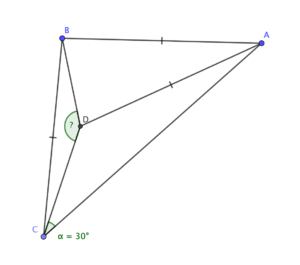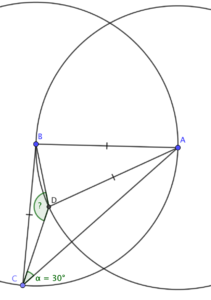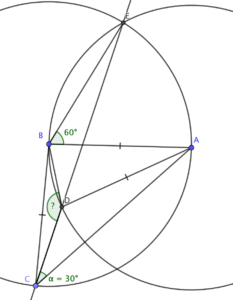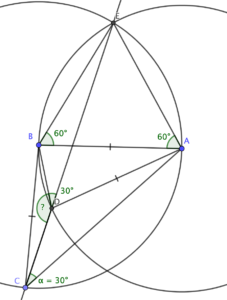A puzzle from @Barney_MT:Find angle BDC

This turns out to be a bit more demanding than I expected. There are spoilers below the line, showing a solution that took rather more time and space than the final polished version does. Spoilers below the line!

When I’ve got isosceles triangles knocking about, I like to add in some circles. Circle theorems, as much as I think they’re a bit of a gimmick at GCSE, are quite useful in geometry puzzles.Now, how do these circles help?

### How about that top intersection?

The way the circles are set up, there’s an equilateral triangle formed by points A, B and the upper intersection of the circles - let’s call that point E.

Here’s the clever bit: C lies on the circle centred at B. Since chord AE subtends 60 degrees at B, it must subtend 30 degrees at C, meaning that E lies on the line CD!That makes it easy.

### Easy?!

Now consider line segment BE, which is a chord of the circle centred at A. It subtends 60 degrees there, so it subtends 30 degrees at D, on the circle’s circumference.And since CDE is a straight line, angle BDC must be 150 degrees.

I’d be curious to learn whether there’s a slicker way of doing that, perhaps one that doesn’t involve circle theorems! Drop me a comment if you can see one.